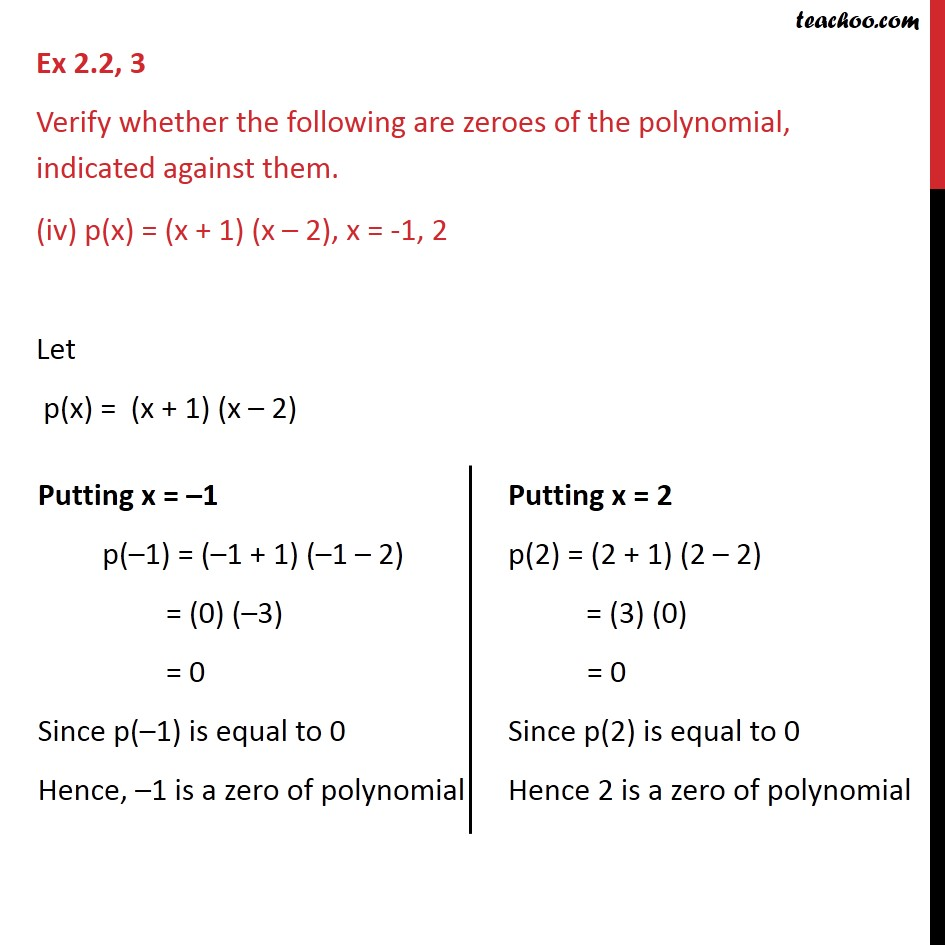Ex 2.2

Chapter 2 Class 9 Polynomials
Serial order wiseLearn in your speed, with individual attention - Teachoo Maths 1-on-1 Class

### Transcript

Ex 2.2, 3 Verify whether the following are zeroes of the polynomial, indicated against them. (iv) p(x) = (x + 1) (x – 2), x = -1, 2 Let p(x) = (x + 1) (x – 2)